##### MOJ
###### eISSN: 2374-6939# Orthopedics & RheumatologyCorrespondence:

Received: January 01, 1970 | Published: ,

Citation: DOI:

# Abstract

Fractal geometry presents a comprehensive mathematical richness capable of describing different aspects of irregular growth phenomena of which the bone structure is an example. In this context we can mention osteoporosis, which is a disease that reaches the bones. It is characterized when the amount of bone mass decreases substantially and develops hollow bones, fine and extremely sensitive, more subject to fractures. It is a variation in the density of the trabecular bone, or a variation in the quantity of trabeculae. Generic modeling of an irregular structure, such as that of the trabeculae of bone, will allow an analytical description of the phenomena resulting from the structure of these trabeculae within the models of bone growth, bone remodeling, osseointegration, etc. In this way, the theoretical models of these phenomena may incorporate the fractal aspects of the trabeculae, explaining them more appropriately, as well as the properties deriving from their geometry in a general way. The objective of this work is to develop a methodology for the measurement of void volume variation and volume variation of trabecular bone material based on fractals.

Keywords: Fractals; Osteoporosis; Remodeling; Osseointegration; Phenomena; Generic; Trabeculae; Bone

# Introduction

The visualization of the bone structure of a patient can be done by different methods, such as: X-rays, tomography, micro-CT, etc. From an image can be made a clinical diagnosis of bone diseases such as osteoporosis, for example. However, these qualitative methods that are commonly used are limited to the orthopedist's visual experience. No quantitative data is provided unless a computerized analysis of these images is performed. A safe diagnosis is the continuing goal of the clinician using the imaging methods outlined above. Since the irregular trabecular structure can be characterized by fractal geometry, in this work we perform a geometric analysis of a bone structure as a way of quantifying the information that can be obtained through an image.

In this work we propose a monofractal model for the trabecular bone structure as a way of quantifying the analyzes made on digitized images. The initial objective of this work is to extract information through the analysis of images that can quantify mathematical quantities defined in the fractal model, depending on the area of ​​observation, such as: effective bone volume, bone volume variation, total trabeculae count, variation of bone density, rate of bone loss, rate of bone remodeling.

In this work we will only present the geometric analyzes made based on the monofractal geometry. We present the fractal study on bone images, where we quantified data related to bone volume variation with the observation scale and the size of the trabecular voids. The objective is to present a research proposal for use in Models of Thermodynamic Potentials Applied to the Study of Phenomena Associated with Porous Matrices as the Bone Trabecular Matrix [1,2].

# Review of the Osteoporosis Problem

Osteoporosis is a disease that strikes the bones. It is characterized when the amount of bone mass decreases substantially and develops hollow bones, fine and extremely sensitive, more subject to fractures. It is part of the normal aging process and is more common in women than in men. The disease progresses slowly and rarely presents symptoms before something of greater gravity happens, such as a fracture, which is usually spontaneous, that is, not related to trauma. If preventative diagnostic tests are not done osteoporosis may go unnoticed until it has greater severity. Osteoporosis can be delayed by preventive measures.

The onset of osteoporosis is linked to the hormonal levels of the body. Estrogen, the female hormone, also present in men, but in lesser amounts, helps maintain the balance between bone loss and bone gain. Women are the most affected by the disease, since at menopause, estrogen levels fall sharply. With this, the bones begin to incorporate less calcium (fundamental in the formation of the bone), becoming more fragile. For every four women, only one man develops this pathology. Although they look like inactive structures, bones change over a lifetime. The body is constantly healing and breaking bones. This process depends on several factors like genetics, good nutrition, maintaining good levels of hormones and regular exercise. The bone cells (osteocytes) are responsible for the formation of collagen, which gives support to the bone. The channels that interconnect the osteocytes allow the calcium, essential for bone formation, to escape from the blood and help form the bone.

The mineral density of calcium is reduced from 65% to 35% when osteoporosis is installed as shown in the Figure 1. The central medullary canal of the bone becomes wider. With the progression of osteoporosis, the bones can become bumpy and brittle. The collagen and mineral deposits are dissolved very quickly and the formation of the bone becomes slower. With less collagen, there are empty spaces in the trabecular structure that weaken the bone, as shown in Figure 2 & 3.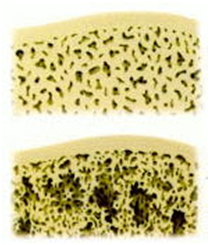Figure 1: Variation of the trabecular structure with the degree of bone calcification.Figure 2: Variation of the size of voids for a: a) trabecular bone with low calcium reduction; b) trabecular bone with high calcium reduction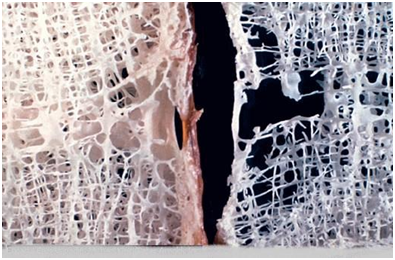Figure 3:Trabecular structure with the appearance of osteoporosis.

Bone remodeling

The amount of bone mass present in the skeleton is the result of formation and reabsorption. This process is directly related to the bodily need to maintain a physiological concentration of ionized calcium in the organic fluids and especially to the need to maintain the structural integrity of the skeleton.

In the normal physiological process, bone resorption and formation are closely related in time, degree and space, so much so that bone formation is only activated after an area of ​​absorption is established. Bone metabolism is influenced by various hormonal, local, behavioral and environmental factors, as well as mechanical, electrical, chemical and magnetic forces. This mechanism is relatively rapid in the trabecular bone and slower in the cortical bone.

Osteoclasts are recruited to the surface (a process called activation) and reabsorb a quantity of mineral, creating a cavity - Howship gap - in the trabecular bone. This phase lasts for about two weeks and is followed by a period of apparent inactivity at the site of resorption. During this phase, the osteoclasts disappear and are replaced by macrophages, whose function is not fully elucidated but appears to be to deposit a substance that initiates cementation. As this process occurs between bone removal and its subsequent replacement, it is called the reversal phase. Upon receipt of a signal, the osteoblasts - cells that synthesize the new matrix - adhere to the surface of the cavity. These cells synthesize collagen and other non-collagenous proteins, which are secreted into the cavity to form the osteoid, a non-mineralized matrix that will later form into new bone. This training phase may take several months to establish. Under normal conditions, the amount of new bone synthesized at each remodeling site is exactly the same as that removed by osteoclasts. Adults are estimated to remodele from 10 to 30% of their bone mass every year. This "preventive maintenance" causes the skeleton to have an average age of about eight years.

Bone remodeling with fractals

The idea of ​​integrating the processes and theories about bone remodeling with fractals theory is to obtain a theoretical model able to demonstrate beyond variation of bone density, trabeculae growth, its direction and size, in relation to its position within the bone.

Using fractal measurements of bone images, in other words, applied the fractal theory, to obtain the fractal dimension, which can be considered a quantitative description of the degree of irregularity of the complex surfaces, on a sequence of images of tomographies, or X-rays, as a function of time, provide formation or resorption characteristics of the trabeculae. With this, it is possible to define a guideline to obtain the specific properties of each trabecula during the process of bone remodeling.

# Fundamentals of Fractal Theory

We are very familiar with Euclidean geometry, where we study the most simple and well-known figures of geometry: straight lines, squares, circles, cones, pyramids, etc. We are accustomed to calculate their length, area, and volume measurements. In this context the idea of ​​dimension is already in our minds. However, many phenomena and forms found in nature cannot be explained along the lines of conventional mathematics, for which a special theory is needed to explain and characterize them, the so-called fractal geometry. In general, fractal geometry is used to describe a geometric object that never loses its structure whatever the distance of sight, or scale. Figure 4 contains drawings of two naturally occurring objects, the boundary (or coastline) of an island (Figure 4a) and a person (Figure 4b). As the contour of the island is enlarged, it is observed that the roughness repeats itself and, by changing the scale, the roughness seems to be the same, that is, the contour of the island is a fractal curve and the island is a self-similar object. The person, however, is not a self-similar object. When various parts of the body are enlarged, complete changes in the shape of objects are observed. The hand does not represent the whole body, the nail does not resemble the hand, and even when observing different parts of the body on the same scale, such as the head and the hand, these parts cannot be said to have similar shapes. This concludes that a person is not a fractal object.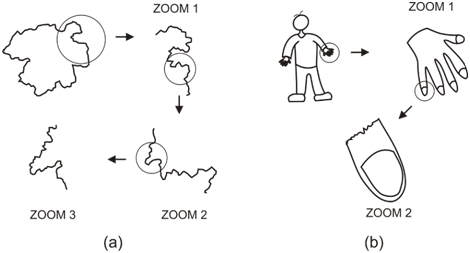Figure 4: Illustration of the boundary of an island, fractal, and a person, not fractal.

Following the observation done above using different scales as illustrated in the Figure 4 we can verify that many objects, phenomena and forms found in nature cannot be explained in the form of conventional mathematics, for which a special theory is necessary to explain and characterize them, the so-called fractal geometry. Both the pattern of cloud formation and the pattern of growth and arrangement of branches and leaves in a tree, for example, can be recreated by means of simple rules of geometric construction, but when executed they can generate structures of admirable complexity, the fractals.

Fractais: they are geometric objects whose Haussdorf-Besicovitch dimension strictly exceeds the topological dimension and have structures in all their scales of expansion, usually with some similarity between them, that is, they are self-invariant geometric objects by scale transformation that they possess fractional dimension exceeding the topological dimension, as shown in the Figure 5.

Invariance by transformation of scale: Is when the parts of an object are similar to the whole that can be by SELF-SIMILARITY (e.g. a pine) or SELF-AFFINITY (e.g. a crack) whose characteristics are: has voids in the structure, has a fractional dimension together the invariance by scale transformation, as shown in the Figure 5 & 6.Figure 5: Mathematical fractal showing its initiator and its seed as basic or fundamental structure of construction.Figure 6: Some types of phenomena that can be modeled by fractal growth theory.

There are basically two types of self-similarity, the statistical and the exact or strict. Statistical self-similarity is the case of the example cited in Figure 4a (the coastline of an island) and in the Figure 5b, as is the case of the trabeculae of a trabecular bone, ie these examples have the same statistical characteristics. In the case of the line of a coast, the roughness of a surface or profile, and in the case of the trabecular bones we observe irregularities that are repeated in a statistically similar way in different magnification scales. In exact or strict self-similarity, one can observe in a part of the fractal object a replica of the whole parenthesis: (Figure 5a), as is the case with the fern leaf.

The term fractal was coined in 1975 by Benoît Mandelbrot, a French mathematician born in Poland, who discovered fractal geometry in the 1970s, from the Latin adjective fractus, from the verb frangere, which means to break. It serves to describe a geometric object that never loses its structure whatever the distance of sight, or scale. Mandelbrot classified his objects of study in this way, for they had a fractional dimension, a non-whole dimension. Fractional dimensions have become a way of quantifying qualities that would otherwise remain without a precise dimension, such as the degree of irregularity or tortuosity of an object. The word fractal above all means self-similar. Self-similarity is the symmetry through the scales, that is, an object has self-similarity if it always presents the same aspect on any scale in which it is observed. If we notice, all orthodox geometric forms lose their structure when they are enlarged or diminished.

It is interesting to note that the body as a whole is not a fractal object, but recent studies have shown with some success that it is possible to characterize certain parts of the body using fractal geometry, such as the branching of the structure of the lung, the thin structure of the neurons and the trabeculae of trabecular bones [3-5].

Measurement of the geometric extension of an object

When we come across an irregular geometric structure such as bone trabeculae, we imagine that it is impossible to quantify data on these structures, except by means of statistical analysis. However, it is intuitively perceived that there is some kind of built-in geometric information that makes us say that such a structure is trabeculae of a bone and not something else. This intuitive visual information can be mathematically identified by fractal geometry. In order to obtain this information in a quantified way, we must use mathematical concepts and equations. The first of these is the idea of ​​a geometric measure, which can be of length, area, volume, etc. In the case of a 2D image of a bone, obtained by X-rays, or microscopy, for example, we can evaluate the area examined by measuring its extent (in the 2D-2D case), which will henceforth be a concept defined as:

${M}_{D}\left(\delta \right)=N\left(\delta \right){\delta }^{d}={M}_{o}$ ,            (1)

Where $N\left(\delta \right)$  is the number of structural elements that recover the object and $d$  is de Euclidean dimension

The object extension, ${M}_{D}$ , depends on the size of the measurement ruler used $\delta =\epsilon {L}_{o}$ .

$Mo\left(\delta /Lo\right)\left(\delta /Lo\right)^\left(d-D\right)$ ,                     (2)

By means of equation (1) a measure is defined as a function of its measurement scale, in addition to the geometric attributes of the topological dimension of the measurement space, the dimension of the unit of measure and the dimension of the measured object.

The functions $F$  that describe the fractal scaling are of the homogeneous type and their differentials are given by:

$dF\left({X}_{j}\right)\equiv \sum _{j=1}^{n}\frac{\partial F}{\partial {X}_{j}}d{X}_{j}$ ,                      (3)

Where ${X}_{j}$  is the variables of this mathematical function for $j=1,2...,n$

The measurement scale is defined in terms of a ruler of size ${l}_{0}$  (used as a unit of measure) and in terms of the maximum size ${L}_{0}$ of the object under measure, as shown by the following equation:

$\lambda =\frac{{L}_{o}}{{l}_{o}}\to \epsilon =\frac{{l}_{o}}{{L}_{o}}$ ,                   (4)

Where $\lambda$ and $\epsilon$  is a scaling factor.

Homogeneous functions also satisfy the Euler theorem which is given by:

$F\left(\lambda {X}_{j}\right)\equiv {\lambda }^{n}\sum _{j=1}^{n}\frac{\partial F}{\partial {X}_{j}}{X}_{j}$ ,                        (5)

from which for mass $M$ , volume $V$  and energy $U$  measurements one can reduce that:

$\frac{\partial F}{\partial {X}_{j}}~\frac{F}{{X}_{j}}⇒\text{\hspace{0.17em}}\text{\hspace{0.17em}}\text{\hspace{0.17em}}\text{\hspace{0.17em}}\frac{dM}{dV}=\frac{M}{V}\text{\hspace{0.17em}}\text{\hspace{0.17em}}\text{\hspace{0.17em}}\text{\hspace{0.17em}};\text{\hspace{0.17em}}\text{\hspace{0.17em}}\text{\hspace{0.17em}}\text{\hspace{0.17em}}\text{\hspace{0.17em}}\text{\hspace{0.17em}}\frac{dU}{dV}=\frac{U}{V}$ ,                         (6)

Mathematically, a fractal can be defined as a geometric sequence $S$  defined by

$S=\sum _{k}{S}_{k}$  onde $k=0,1,2...$                         (7)

Where ${S}_{k}$  is the subsequence, represented in the Euclidean space when the measure of its geometric extension, given by the series ${M}_{d}\left({\delta }_{k}\right)$ , satisfies the following Hausdorf-Besicovitch condition:

${M}_{d}\left({\delta }_{k}\right)=\sum _{k}\gamma \left(d\right){\delta }_{k}^{d}=N\left({\delta }_{k}\right)\underset{\delta \to 0}{\gamma }\left(d\right){\delta }_{k}^{d}=\left\{\begin{array}{l}0\to Dd\end{array}$                   (8)

Being $\gamma \left(d\right)$  the geometric factor of the unit elements (or seed) of the geometrically represented sequence, $\delta$  is the size of the elements, used as the standard unit of measure of the geometric extension of the spatial representation of the sequence, $N\left(\delta \right)$ is the number of elementary units (or seeds) Spatial representation of the sequence on a given scale, d is the dimension of the unitary elements and $D$ is the Hausdorff-Besicovitch dimension.

Relationship between fractal geometry and euclidean

Some irregular structures are considered as fractals with self-similarity or statistical self-affinity. A fractal is an object whose measure of its geometric extent depends on the measuring ruler used. This object is defined as having one Hausdorff-Besicovitch dimension.

According to Figure 7 it is observed that a fractal always exceeds a Euclidean dimension and has a fault in the dimension immediately above which it is immersed. Let's look at the examples: a Cantor curve fractal has a dimension in the interval 0 £ D £ 1, according to Figure 7, it exceeds one point but is not a straight line. A rough profile has a fractal dimension in the interval 1 £ D £ 2, according to Figure 7, it exceeds a straight line, but does not reach a plane. A rough surface has fractal dimension in the interval 2 £ D £ 3, and according to Figure 7, it exceeds a plane but does not reach a solid.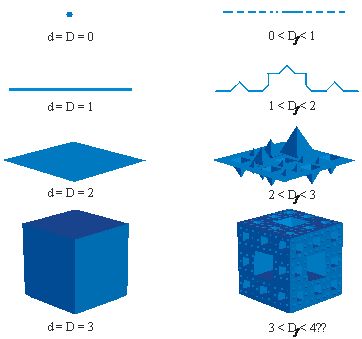Figure 7: Euclidean geometry x Fractal geometry.

But what do fractals serve in practice?: The fractals serve to describe mathematically irregular structures, which are not possible by the basic elements, Point, Line, Plane and Space of Euclidean geometry. Note the following geometric modeling shown in Figure 8. This geometric modeling example used flat figures such as circle, rectangle, and triangle to geometrically represent some more elaborate figures such as a person, a dog, and a car. On the other hand, in Figure 9, it can be seen that figures of non-Euclidean geometries such as cracks, clouds, lightning, etc., cannot be easily described by simple regular forms of Euclidean geometry. In this case it is necessary to resort to more complex geometric models such as the fractal models, as we will see next.

# Fractal Modeling of the Trabecular Structure

Fractal geometry presents a comprehensive mathematical richness capable of describing different aspects of irregular growth phenomena of which the bone structure is an example. It is believed that it is possible to model generically an irregular structure, such as that of the bone trabeculae, this will allow an analytical description of the phenomena arising from the structure of these trabeculae within the bone growth, bone remodeling, osseointegration and healing models. In this way the theoretical models of these phenomena can incorporate the fractal aspects of the trabeculae explaining them more appropriately as well as the properties deriving from their geometry in a general way.

Discretization of a bone by the box-counting method

Consider the following trabecular structure, which can be considered a fractal (or multifractal), as being representative of a bone structure, as shown in Figure 10.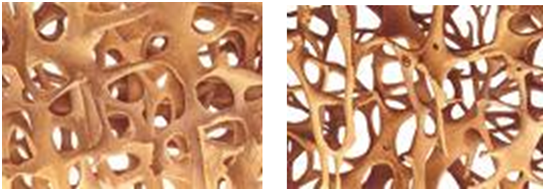Figure 10: Geometric differentiation of trabecular structure with variation of calcium content.

On these models an initial mesh is constructed and by means of a process of consecutive refinements, of order, i, that is, we proceed to the following construction, as shown in Figure 11. For this, several steps of mesh refining are adopted, converging the model to the result.Figure 11: Measure of the trabecular matrix by the Box-Counting using the concept of ruler and length.

In each iteration $i$  the mesh is subdivided into a smaller mesh opening (or spacing) of length ${l}_{i}$ . Rectangular mesh cells or reticles that do not intersect the trabecula are considered empty regions or cells or absent segments of trabecula, where

${l}_{i}=\frac{{L}_{0}}{{a}^{i}}.$           (9)

Where ${l}_{i}$  is the width of the mesh and ${L}_{0}$  is the apparent horizontal size in the x-direction and $a$  is the mesh reduction factor in the horizontal direction and ${h}_{i}$  is the height of the mesh

${h}_{i}=\frac{{H}_{o}}{{b}^{i}}.$          (10)

Where ${h}_{i}$  is the height of the mesh and ${H}_{0}$  is the apparent vertical size in the y-direction and $b$  is the mesh reduction factor in the vertical direction.

The mesh elements that do not intersect the trabeculae are considered empty regions, absent from trabeculae. Thus, we can write the total irregular area of ​​the structure as,

$A=N{A}_{\mathrm{max}}$          (11)

Where, $N$  is the total number of non-empty elements, and ${A}_{\mathrm{max}}$  is the area of ​​an element.

In the case of this discretization, hexahedral elements were used, the area of ​​an element can be calculated in the following way, for each refining step,

${A}_{\mathrm{max}}={l}_{i}{h}_{i}$            (12)

Approximation of the monofractal model: The Monofractal modeling consists of admitting that each element of the mesh that is not empty or totally filled will have a fixed amount of trabecular structure. Considering the following trabecular structures as being representative of a normal bone structure and another one of osteoporosis, as shown in Figure 12, showing a trabecular bone. Considering that each element the trabecula area is that of a rectangle minus the area of ​​an ellipse inscribed in this rectangle, as shown in the Figure 13, one has that:

${A}_{\mathrm{min}}={A}_{rec\mathrm{tang}le}-{A}_{elípse}$          (13)Figure 12: Estrutura trabecular normal (a) e estrutura trabecular com osteoporose (b).

Being, ${A}_{\mathrm{min}}$  the area of ​​the intermediate element. Figure 14 illustrates this schematization of ellipses in rectangles for the normal trabecular structure for the first mesh adopted.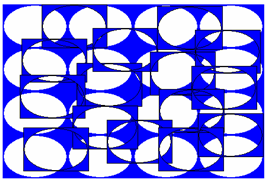Figure 13: Geometric model of a trabecular structure with the diameters of the voids constant.Figure 14: Monofractal approximation scheme of a normal trabecular structure (a), approximate trabecular structure (b).

As the mesh is refined, the model is closer to the structures of the real trabeculae. Figure 15 shows the first refining of this mesh. It is noted that the mesh with the first refining, Figure 15b is closest to the trabecular structure shown in Figure 15a. The mesh refiners are run until the discretized model can accurately describe the actual model.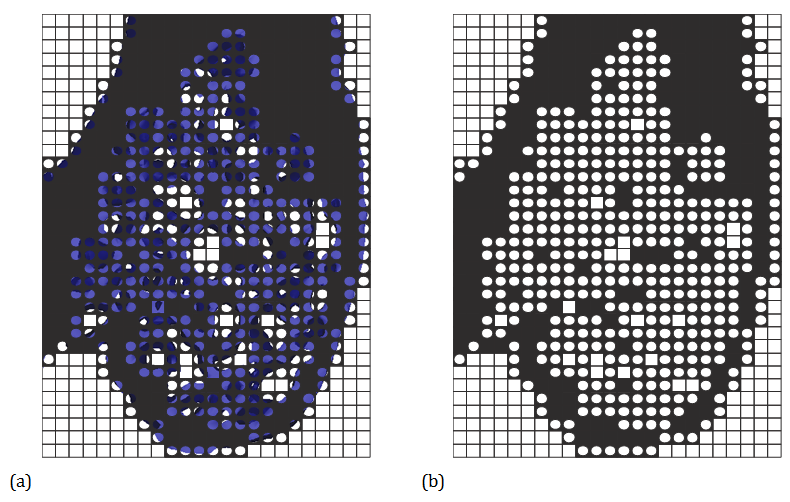Figure 15: Refining the mesh of the monofractal approach scheme.

The values ​​of the area of ​​a rectangle and an ellipse are known. Substituting them into equation ( 13), we arrive at,

${A}_{\mathrm{min}}={l}_{i}{h}_{i}-\pi {l}_{i}{h}_{i}$        (14)

Substituting equations ( 9) and ( 10) into equation ( 14), we obtain

${A}_{\mathrm{min}}=\frac{{L}_{o}}{{a}^{i}}\frac{{H}_{o}}{{b}^{i}}\left(1-\pi \right)$        (15)

A particular case of equation ( 15) is observed when the horizontal refining follows the same rule as the vertical refining, and therefore, ${a}^{i}={b}^{i}={r}^{i}$  being ${r}^{i}$  a general reduction factor of the mesh. For this particular case, equation (13) can be rewritten using equations ( 11),( 12) and ( 13) of the form,

$A=\frac{{L}_{o}{H}_{o}}{{r}^{2i}}\left[{N}_{\mathrm{max}}+{N}_{\mathrm{min}}\left(1-\pi \right)\right]$            (16)

The only unknown variables of equation (16) are the values ​​of ${N}_{\mathrm{max}}$  and ${N}_{\mathrm{min}}$ , which can be easily found by counting the numbers of fully filled elements and intermediate elements.

Calculation of the effective volume and rate of variation of bone volume: Considering that the total irregular volume of the trabecular structure is given by:

$V=N{V}_{\mathrm{min}}$                         (17)

Where N is the total number of filled boxes and ${V}_{\mathrm{min}}$  is the unit volume of a box, given by:

${V}_{\mathrm{min}}={V}_{square}-{V}_{elipse}$              (18)

Or

${V}_{\mathrm{min}}={l}_{i}{h}_{i}-\pi {l}_{i}{h}_{i}$ ,           (19)

Or

${V}_{\mathrm{min}}={l}_{i}{h}_{i}\left(1-\pi \right)$ ,          (20)

Then replacing ( 9) and ( 10) in ( 20) we get:

${V}_{\mathrm{min}}=\frac{{L}_{o}}{{a}^{n}}\frac{{H}_{o}}{{b}^{n}}\left(1-\pi \right)$ ,     (21)

Considering $a=b$  we have:

${V}_{\mathrm{min}}=\frac{{L}_{o}{H}_{o}}{{a}^{2n}}\left(1-\pi \right)$ ,        (22)

and still

$V=N{V}_{\mathrm{min}}=N\left({V}_{square}-{V}_{elipse}\right)$ ,            (23)

Therefore,

$V=\frac{N{L}_{o}{H}_{o}}{{a}^{2n}}\left(1-\pi \right)$ ,                (24)

Knowing that each structure of minimum size has a volume given by the unit model.

${V}_{\mathrm{min}}={l}_{o}{}^{2}-\pi {\left(\frac{{l}_{o}}{2}\right)}^{2}={l}_{o}{}^{2}\left(1-\frac{\pi }{4}\right)$ ,             (25)

Thus, the total volume of the analyzed region has, $N$  unit volumes, then

$V=N{V}_{\mathrm{min}}=N\left({V}_{square}-{V}_{elipse}\right)$ ,               (26)

Substituting ( 25) into ( 26) we have:

$V\left({L}_{0},D\right)=\frac{N{L}_{o}{H}_{o}}{{a}^{2n}}\left(1-\pi \right)=N{l}_{o}{}^{2}\left(1-\frac{\pi }{4}\right)$ ,             (27)

Now the mathematical problem to be solved in the case of any curve is to write the number, N, and dimensions of the coating boxes in terms of quantities that can be easily measured.

Let us now assume that in ( 27) the fractal exponent, D e can be considered as a single exponent, because the object is monofractal, just as in equation ( 8) we therefore have the number of cells in the x and y direction, which intercept the curve on any horizontal slice can be written as:

$N={\left(\frac{{l}_{0}}{{L}_{0}}\right)}^{-D}$ ,                      (28)

Therefore, the fractal volume of the matrix is ​​given by:

$V=\frac{{L}_{o}{H}_{o}}{{a}^{2n}}\left(1-\pi \right){\left(\frac{{l}_{0}}{{L}_{0}}\right)}^{-D}=\left(1-\frac{\pi }{4}\right){\left(\frac{{l}_{0}}{{L}_{0}}\right)}^{-D}{l}_{0}^{2}$ ,                       (29)

This equation gives the real bone volume as a function of the fractal dimension.

Mass measurement of a cell

Now inside the slices, for each cell, we will also classify the structures of the trabeculae and the empty regions, as follows.

A trabecula may have different degrees of irregularities each classified in the spectrum of Figure 16. In this way a single trabecular structure may present different dimensions of capacity, as shown in Figure 16.Figure 16: Spectrum of the capacity of the box capacity in 2D.

Consider that each slice also contains cells with different degrees of irregularities. And that these degrees can be represented by different capacity exponents, according to the spectrum of Figure 16. Thus, each cell in a slice has a fill probability that is revealed at each refinement of the mesh. Thus, with each new refinement of the mesh the capacity dimension of the cells can vary because for a more resolved mesh each cell can show new details of the segment that was previously not perceived in the refinement of the previous mesh in such a way that the capacity exponent, a, varies, according to Figure 16. This classification of capacity exponent $\alpha$  can be made constructing an algorithm specially developed by using a data bank of basic images as exemplified in the Figure 16.

For each structure contained in a slice of the mesh in this mesh we assign a fraction of the mass p of the segment of the previous mesh. The masses of these segments are not equal if p ≠ 1.

Similarly for each segment a numbering can be given that corresponds to its refinement order ranging from 0 ≤ i ≤ N - 1. This other binary representation of the integer i will characterize the segment to give us the history of segment refinements In the sense that the number of zeros corresponds to the number of empty regions and the number of ones corresponds to the number of segments of non-empty cracks of each refinement. For example, by analogy with the Cantor fractal of Figure 17, for n = 3 we have 8 segments and segment 3 whose binary representation is 011 resulted from the refinement of a first empty segment on the left followed by two segments of crack Right, as shown in Figure 17. The mass of this segment will then be (1 - p) p2. Note that there are other segments with this same mass that can be counted by combinatorial analysis. For example, segment 6 whose binary representation is 110. In fact, for n = 3 there are 3 segments with mass p (1 - p)2.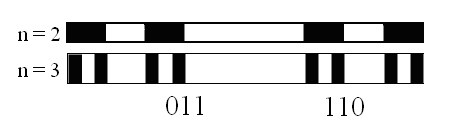Figure 17: Spectrum of the capacity of a slice in 1D.

Therefore, in this way, using genetic algorithm, it is possible reconstruct a bone in computer simulation.

Capacity of a cell

Therefore, the local probability p that a cell contains a fraction of points ${N}_{o}/{N}_{n}$  is given by:

$p=\frac{{N}_{o}}{{N}_{n}}={\left(\frac{{l}_{o}}{{l}_{n}}\right)}^{-\alpha }$  ,               (30)

Where $\delta$  is the box size. So the probability that a cell appears in an empty pixel is given by:

$q=1-p=1-\frac{{N}_{o}}{{N}_{n}}=1-{\left(\frac{{l}_{o}}{{l}_{n}}\right)}^{-\alpha }$ ,                    (31)

In general a segment of crack whose binary representation has u zeros has mass

${\rho }_{k}={p}^{u}{\left(1-p\right)}^{{N}_{n}-u}$ ,                   (32)

and there are

$\left(\begin{array}{l}{N}_{n}\\ u\end{array}\right)=\frac{n!}{u!\left({N}_{n}-u\right)!}$ ,                     (33)

Segments of crack with this same mass.

It is necessary to add on all the slices to obtain the mass all of the slices:

$\sum _{u=0}^{N}\left(\begin{array}{l}{N}_{n}\\ u\end{array}\right){\rho }_{k}=\sum _{r=0}^{N}\frac{n!}{u!\left({N}_{n}-u\right)!}{p}^{u}{\left(1-p\right)}^{{N}_{n}-u}$ ,                   (34)

This combinatorial mathematical description of the trabeculae can be used to obtain a multifractal spectrum analysis of the bone.

Discretization of a bone by the slice method

We know that any fractal line, corresponding to the excess of a line and to the lack of a plane. Like each slice, the number of filled boxes is analogous to the Cantor fractal. So if we consider a slice to be a straight segment, and its boxes as points, we see that at the intersection with the trabecula structure, the slice exceeds one point but lacks a straight line. For, more than one box is filled, yet the slice is not fully filled. This allows us to write the number of cells in the x and y directions, which intercept the curve in a horizontal and vertical slice respectively, as shown in the Figure 18, such as:Figure 18: Counting of trabecular structures using the slice method.

Let's slice the mesh so that we get several slices corresponding to a fractal line analogous to a Cantor fractal, but with irregular distribution of segments. The trabecula structure can be discretized in the x and y directions by horizontal size slices $\left({L}_{o},{l}_{n}\right)$  and by vertical size slices $\left({h}_{n},{H}_{o}\right)$  as shown in Figure 19.Figure 19: Discretization of a fractal curve by means of horizontal and vertical slices.

In iteration $n$ , for each horizontal slice of the mesh, we have a number of cells equal to:

${N}_{Hn}=\frac{{L}_{o}}{{l}_{n}}$ ,                         (35)

and, for each vertical slice of the mesh, we have the number of cells a:

${N}_{Vn}=\frac{{H}_{o}}{{h}_{n}}$ ,                            (36)

Therefore, the whole mesh will have a total number of:

${N}_{{T}_{n}}={N}_{Hn}.{N}_{Vn}=\frac{{L}_{0}{H}_{0}}{{l}_{n}{h}_{n}}=\frac{{A}_{0}}{{A}_{n}}$ ,                   (37)

Cells contained in this mesh.

Monofractality of horizontal and vertical slices : Let us now assume that the exponent ${\alpha }_{xi}$  can be considered a single exponent, because the curve is monofractal, in the same way ${\alpha }_{yj}$ , we have, therefore,

${\alpha }_{xi}={\alpha }_{x};\text{\hspace{0.17em}}0\le {\alpha }_{x}\le 1$ ,                   (38)

And

${\alpha }_{yj}={\alpha }_{y};\text{\hspace{0.17em}}0\le {\alpha }_{y}\le 1$  ,                  (39)

Being self-related behavior, we have,

${\alpha }_{x}\ne {\alpha }_{y}$ ,                             (40)

The number of cells in the x-direction, which intercept the curve in any horizontal slice can be written as:

${n}_{H}={\left(\frac{{L}_{o}}{{l}_{o}}\right)}^{{\alpha }_{x}}0\le {\alpha }_{x}\le 1$ ,                  (41)

Where ${\alpha }_{x}$  is a fractional exponent extracted directly from the fractal analysis of the image, in analogue way to fractal dimension for the horizontal slice.

However in the y direction, the number of cells that intercept the curve in any vertical slice is unique and can be written as:

${n}_{V}={\left(\frac{{H}_{o}}{{h}_{o}}\right)}^{{\alpha }_{y}}0\le {\alpha }_{y}\le 1$ ,                   (42)

Where ${\alpha }_{y}$  is a fractional exponent extracted directly from the fractal analysis of the image, in analogue way to fractal dimension for the vertical slice.

Multifractality of horizontal and vertical slices: Let us now assume that the exponent ${\alpha }_{xl}$  are different exponent for each slice, because the curve is multifractal, in the same way ${\alpha }_{ym}$ , we have, therefore,

${n}_{Hl}={\left(\frac{{L}_{o}}{{l}_{n}}\right)}^{{\alpha }_{xl}}0\le {\alpha }_{xl}\le 1\text{\hspace{0.17em}}\text{\hspace{0.17em}}\text{\hspace{0.17em}};\text{\hspace{0.17em}}\text{\hspace{0.17em}}l=1,2,...,\frac{{H}_{o}}{{h}_{n}}$ ,                   (43)

Where ${\alpha }_{xl}$  is a fractional exponent extracted directly from the fractal analysis of the image, in analogue way to fractal dimension for the horizontal slice.

${n}_{Vm}={\left(\frac{{H}_{o}}{{h}_{n}}\right)}^{{\alpha }_{ym}}0\le {\alpha }_{ym}\le 1\text{\hspace{0.17em}}\text{\hspace{0.17em}}\text{\hspace{0.17em}};\text{\hspace{0.17em}}\text{\hspace{0.17em}}m=1,2,...,\frac{{L}_{o}}{{l}_{n}}$ ,                (44)

Where ${\alpha }_{ym}$  is a fractional exponent extracted directly from the fractal analysis of the image, in analogue way to fractal dimension for the vertical slice.

Then

${N}_{Hn}=\left(\frac{{L}_{o}}{{l}_{n}}\right)={n}_{Hl}{}^{\frac{1}{{\alpha }_{xl}}}$ ,                      (45)

And

${N}_{Vn}=\left(\frac{{H}_{o}}{{h}_{n}}\right)={n}_{Vm}{}^{\frac{1}{{\alpha }_{ym}}}$ ,                         (46)

For it is always possible to find exponents ${\alpha }_{xl},{\alpha }_{ym}$  that satisfy the above relations. From ( 9) and ( 10) we have:

${N}_{Hn}=\left(\frac{{L}_{o}}{{l}_{n}}\right)={n}_{Hl}{}^{\frac{1}{{\alpha }_{xl}}}={a}^{n}$ ,                    (47)

And

${N}_{Vn}=\left(\frac{{H}_{o}}{{h}_{n}}\right)={n}_{Vm}{}^{\frac{1}{{\alpha }_{ym}}}={b}^{n}$ ,     (48)

Then

${n}_{Hl}={a}^{n{\alpha }_{xl}}$ ,          (49)

Or

${n}_{Vm}={b}^{n{\alpha }_{ym}}$ ,        (50)

Therefore, the total number of cells is given by:

${N}_{{T}_{n}}={N}_{Hn}.{N}_{Vn}={n}_{Hl}{}^{\frac{1}{{\alpha }_{xl}}}{n}_{Vm}{}^{\frac{1}{{\alpha }_{ym}}}$ ,                     (51)

Discretization of a bone by the orthogonal projection method

In order to obtain the projections of the fractal curves on the orthogonal axes, it is necessary to add all the possible slices of the previous item, especially the curve range, both horizontally and vertically, within the size grid (Lo, Ho).

Note that as a mandatory number of slices covers the entire object (Figure 19) in the size grid interval (Lo, Ho). Therefore, the total number of cells intercepting the object, counted horizontally, must be equal to the number of cells that intercept the object vertically, which leads to the relation

NTH = NTV,          (52)

Monofractality of the orthogonal projections: Similarly for monofractals, adding all possible slices both horizontally and vertically within the size grid (Lo, Ho), we have that the total number of horizontal and vertical slices are given respectively by:

${N}_{TH}=\sum _{i=1}^{{H}_{o}/{h}_{o}}{n}_{Hi}=\sum _{i=1}^{{H}_{o}/{h}_{o}}{\left(\frac{{L}_{o}}{{l}_{o}}\right)}^{{\alpha }_{x}}=\frac{{H}_{o}}{{h}_{o}}{\left(\frac{{L}_{o}}{{l}_{o}}\right)}^{{\alpha }_{x}}$ ,                  (53)

${N}_{TV}=\sum _{j=1}^{{L}_{o}/{l}_{o}}{n}_{Vi}=\sum _{j=1}^{{L}_{o}/{l}_{o}}{\left(\frac{{H}_{o}}{{h}_{o}}\right)}^{{\alpha }_{y}}=\frac{{L}_{o}}{{l}_{o}}{\left(\frac{{H}_{o}}{{h}_{o}}\right)}^{{\alpha }_{y}}$ ,                  (54)

Analogously to the previous case we have:

NTH =NTV,                (55)

Thus the total number in this case is given by:

$\frac{{H}_{o}}{{h}_{o}}{\left(\frac{{L}_{o}}{{l}_{o}}\right)}^{{\alpha }_{x}}=\frac{{L}_{o}}{{l}_{o}}{\left(\frac{{H}_{o}}{{h}_{o}}\right)}^{{\alpha }_{y}}$ ,                (56)

What gives the following relation between the horizontal and vertical scales

${\left(\frac{{L}_{o}}{{l}_{o}}\right)}^{{\alpha }_{x}-1}={\left(\frac{{H}_{o}}{{h}_{o}}\right)}^{{\alpha }_{y}-1}$ ,           (57)

For the case of monofractal this is the necessary relation to be used in the scaling equations of lengths, areas or trabecular volume given in (29).

Multifractality of the orthogonal projections: For the multifractal case we will have that the total number of horizontal and vertical slices are given respectively by:

${N}_{TH}=\sum _{i=1}^{{H}_{o}/{h}_{o}}{n}_{Hi}=\sum _{i=1}^{{H}_{o}/{h}_{o}}{\left(\frac{{L}_{o}}{{l}_{o}}\right)}^{{\alpha }_{xi}}$ ,      (58)

${N}_{TV}=\sum _{j=1}^{{L}_{o}/{l}_{o}}{n}_{Vj}=\sum _{j=1}^{{L}_{o}/{l}_{o}}{\left(\frac{{H}_{o}}{{h}_{o}}\right)}^{{\alpha }_{yj}}$ ,          (59)

Logo

${N}_{TH}=\sum _{i=1}^{{H}_{o}/{h}_{o}}{n}_{Hi}=\sum _{j=1}^{{H}_{o}/{h}_{o}}{n}_{Vj}$ ,                  (60)

Or

$\sum _{i=1}^{{H}_{o}/{h}_{o}}{\left(\frac{{L}_{o}}{{l}_{o}}\right)}^{{\alpha }_{xi}}=\sum _{j=1}^{{L}_{o}/{l}_{o}}{\left(\frac{{H}_{o}}{{h}_{o}}\right)}^{{\alpha }_{yj}}$ ,               (61)

For the case of multifractal this is the necessary relation to be used in the scaling equations of lengths, areas or trabecular volume given in (29).

Horizontal trabecular counting

Counting the structures within each horizontal slice we have:

${n}_{Hi}={\left(\frac{{L}_{o}}{{l}_{o}}\right)}^{{\alpha }_{xi}}0\le {\alpha }_{xi}\le 1$ ,                  (62)

Therefore sweeping all the area or volume of the region analyzed we have:

${N}_{TV}=\sum _{j=1}^{{L}_{o}/{l}_{o}}{n}_{Vj}=\sum _{j=1}^{{L}_{o}/{l}_{o}}{\left(\frac{{H}_{o}}{{h}_{o}}\right)}^{{\alpha }_{yj}}=\frac{{L}_{o}}{{l}_{o}}{\left(\frac{{H}_{o}}{{h}_{o}}\right)}^{{\alpha }_{y}}$ ,           (63)

Vertical trabecular counting

Counting the structures within each vertical slice we have:

${n}_{Vj}={\left(\frac{{H}_{o}}{{h}_{o}}\right)}^{{\alpha }_{yi}}0\le {\alpha }_{yi}\le 1$ ,           (64)

Therefore sweeping all the area or volume of the region analyzed we have:

${N}_{TH}=\sum _{i=1}^{{H}_{o}/{h}_{o}}{n}_{Hi}=\sum _{i=1}^{{H}_{o}/{h}_{o}}{\left(\frac{{L}_{o}}{{l}_{o}}\right)}^{{\alpha }_{x}}=\frac{{H}_{o}}{{h}_{o}}{\left(\frac{{L}_{o}}{{l}_{o}}\right)}^{{\alpha }_{x}}$ ,           (65)

Total counting in whole volume

We know that the two previous counts (horizontal and vertical in 2D and a third for the 3D model) must have the same result, so we can match these values ​​by getting

${N}_{TH}=\sum _{i=1}^{{H}_{o}/{h}_{o}}{n}_{Hi}=\sum _{j=1}^{{H}_{o}/{h}_{o}}{n}_{Vj}={N}_{TV}$ ,     (66)

From where the total number of irregular structures is easily extracted, obtaining

$N=\frac{{H}_{o}}{{h}_{o}}{\left(\frac{{L}_{o}}{{l}_{o}}\right)}^{{\alpha }_{x}}=\frac{{L}_{o}}{{l}_{o}}{\left(\frac{{H}_{o}}{{h}_{o}}\right)}^{{\alpha }_{y}}\text{\hspace{0.17em}}$ ,        (67)

For the case of monofractal this is the necessary relation to be used in the scaling equations of lengths, areas or trabecular volume given in (29).

Autosimilarity of the counting

For the sake of simplicity we can consider that the structures do not have a preferential direction, they are self-similar, so we can do:

${H}_{o}={L}_{o}\text{\hspace{0.17em}}\text{\hspace{0.17em}}\text{\hspace{0.17em}}\text{\hspace{0.17em}}e\text{\hspace{0.17em}}\text{\hspace{0.17em}}\text{\hspace{0.17em}}{h}_{o}={l}_{o}$ ,          (68)

and therefore,

$N={\left(\frac{{L}_{o}}{{l}_{o}}\right)}^{1+\alpha }$ ,                     (69)

For the case of monofractal this is the necessary relation to be used in the scaling equations of lengths, areas or trabecular volume given in (29).

Fractal scaling of the bone volume

Starting from the unit volume considered in the geometric model

$V=N{l}_{o}{}^{2}\left(1-\frac{\pi }{4}\right)$ ,                        (70)

The Real Volume of Bone Mass can be written by substituting ( 69) into ( 70) and obtaining:

$V={\left(\frac{{L}_{o}}{{l}_{o}}\right)}^{1+\alpha }{l}_{o}{}^{2}\left(1-\frac{\pi }{4}\right)$ ,           (71)

We can also write this real volume in terms of the apparent volume given by:

${V}_{o}={L}_{o}{}^{2}$ ,        (72)

and obtain

$V=\frac{{V}_{o}}{{L}_{o}{}^{2}}{\left(\frac{{L}_{o}}{{l}_{o}}\right)}^{1+\alpha }{l}_{o}{}^{2}\left(1-\frac{\pi }{4}\right)$ ,                (73)

Or

$V={V}_{o}\frac{{l}_{o}{}^{2}}{{L}_{o}{}^{2}}{\left(\frac{{L}_{o}}{{l}_{o}}\right)}^{1+\alpha }\left(1-\frac{\pi }{4}\right)$ ,            (74)

Therefore,

$V={V}_{o}{\left(\frac{{L}_{o}}{{l}_{o}}\right)}^{\alpha -1}\left(1-\frac{\pi }{4}\right)$ ,                (75)

This is the equation that according to the proposed monofractal model describes the actual bone volume of the irregular structures of the trabecular structure in an area of ​​observation delimited by an apparent volume ${V}_{o}={L}_{o}{}^{2}$ .

Bone loss rate

To obtain a mathematical formulation for bone loss or gain as a function of time, it is enough to derive the previous result in relation to time and obtain:

$\frac{dV}{dt}=\left(1-\alpha \right){V}_{o}{}^{1-\alpha }{\left(\frac{{L}_{o}}{{l}_{o}}\right)}^{2\alpha -1}\left(1-\frac{\pi }{4}\right)\frac{d{V}_{o}}{dt}$ ,               (76)

Multiplying everything by bone density r we have:

$\frac{dm}{dt}=\rho \left(1-\alpha \right){V}_{o}{}^{1-\alpha }{\left(\frac{{L}_{o}}{{l}_{o}}\right)}^{2\alpha -1}\left(1-\frac{\pi }{4}\right)\frac{d{V}_{o}}{dt}$ ,                    (77)

Note that the rate of bone loss can be calculated in terms of the rate of change in apparent bone volume.

Variation of bone density

Knowing that we have:

$dM=\rho dV$ ,           (78)

Considering that the same bone mass is analyzed we have;

$dM=d{M}_{o}$ ,                    (79)

Then

$\rho dV={\rho }_{o}d{V}_{o}$ ,               (80)

So

${\rho }_{o}=\rho \frac{dV}{d{V}_{o}}$ ,                                 (81)

From ( 75) we have to:

$\frac{dV}{d{V}_{o}}=\left(1-\frac{\pi }{4}\right)\frac{{l}_{o}{}^{2}}{{L}_{o}{}^{2}}{\left(\frac{{L}_{o}}{{l}_{o}}\right)}^{1+\alpha }$ ,                          (82)

Therefore,

$\rho ={\rho }_{o}\frac{4}{\left(4-\pi \right)}{\left(\frac{{L}_{o}}{{l}_{o}}\right)}^{1-\alpha }$ ,                     (83)

Note that the actual bone density can be calculated in terms of the apparent density of the bone.

Bone remodellig rate

Knowing that:

${V}_{o}=\frac{{M}_{o}}{{\rho }_{o}}$ ,                  (84)

Considering that a same bone volume is analyzed we have;

${V}_{o1}={V}_{o2}$ ,               (85)

Then

$\frac{{M}_{o1}}{{\rho }_{o1}}=\frac{{M}_{o2}}{{\rho }_{o2}}$ ,                (86)

From ( 75) we have to:

$V={V}_{o}{\left(\frac{{L}_{o}}{{l}_{o}}\right)}^{\alpha -1}\left(1-\frac{\pi }{4}\right)$ ,                       (87)

Then

${V}_{1}\left(\frac{4}{4-\pi }\right){\left(\frac{{L}_{o}}{{l}_{o1}}\right)}^{1-{\alpha }_{1}}={V}_{2}\left(\frac{4}{4-\pi }\right){\left(\frac{{L}_{o}}{{l}_{o2}}\right)}^{1-{\alpha }_{2}}$ ,                  (88)

And

${V}_{1}{\left(\frac{{L}_{o}}{{l}_{o1}}\right)}^{1-{\alpha }_{1}}={V}_{2}{\left(\frac{{L}_{o}}{{l}_{o2}}\right)}^{1-{\alpha }_{2}}$ ,              (89)

Multiplying everything by r we have:

$\rho {V}_{1}{\left(\frac{{L}_{o}}{{l}_{o1}}\right)}^{1-{\alpha }_{1}}=\rho {V}_{2}{\left(\frac{{L}_{o}}{{l}_{o2}}\right)}^{1-{\alpha }_{2}}$ ,                        (90)

All these results of these calculations are mathematical equations that can represent the characteristics of a trabecular structure according to the monofractal and multifractal models. What remains is its application in practice to verify its validity in the geometric and physical characterization of the processes that take place in the bone matrix in the osteoporosis and healing phenomena.

# Materials and Methods

Based on the following figures obtained in a microscopic analysis of a trabecular structure, as shown in the Figure 20, we will use the box-counting method to analyze these structures. For this first analysis we used Fractal Vision Software to obtain the fractal dimension of these structures.Figure 20: Change in the shape of the trabecular structure with the calcium content in a case of osteoporosis.

We used the microscopic analysis shown in Figure 21, to apply the Box Counting technique with half mesh reduction at each count as shown in the Figure 22-24 [6-12].Figure 21: Trabecular structure in two different calcification conditions.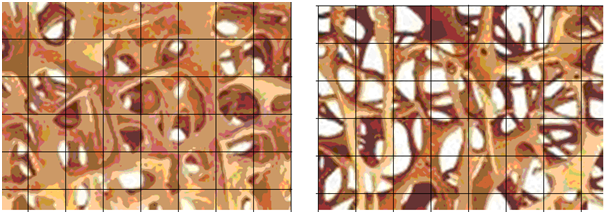Figure 22: Counting boxes using a mesh size of δ.Figure 23: Counting boxes using a mesh of size δ/2.Figure 24: Counting boxes using a mesh size of δ/4.

# Results and Discussion

In this section we describe the fractal characterization of some bone structures to verify the models presented in item 5.0 of this article.

Measures of the fractional dimensions of the bone dies

Applying the Box-Counting method to the case shown in Figure 22-24 the results shown in Table 1 & 2.

 Size Box Fractal Dimension Number of Structural Elements Normal - 1 Osteoporótico - 1 Normal - 1 Osteoporótico - 2 2 1,705,463 1,355,219 2,55835949 3,26133581 4 1,602,282 1,128,430 4,77950099 9,21870435 6 1,600,730 1,225,713 8,99062955 17,6039469 8 1,863,938 1,269,649 14,0154581 48,2284988

Table 1: Fractional Measures of the Case – 1.

 Size Box Fractal Dimension Number of Structural Elements Normal - 2 Osteoporótico - 2 Normal - 2 Osteoporótico - 2 2 15,483,350 180,088 29,247,940 34,843,366 4 13,984,410 17,444 69,493,691 112,262,485 6 12,724,950 181,622 97,767,290 258,996,945 8 13,314,510 18,006 159,374,952 422,773,194

Table 2: Fractional Measures of the Case – 2.

Graphing the count of boxes obtained in the structures analyzed we have the results shown in Figure 25 & 26.Figure 25: Variation of the fractal dimension with the measurement scale.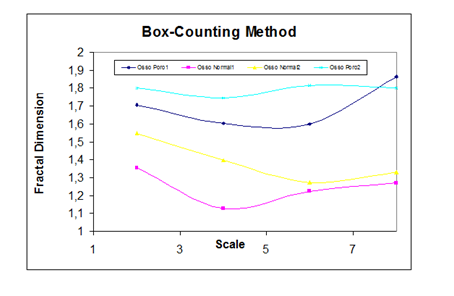Figure 26: Variation of the fractal dimension with the measurement scale.

Variation of Volume with the sizes of voids

By calculating the variation of bone volume with the apparent length, L0 we have the result shown in Figure 27.Figure 27: Chart of effective bone volume.

Analyzing the volumetric fraction of the bones as a function of the size of the observed voids, we have the graph of Figure 28 The analyzes provided a volume equation given by:

$V={V}_{o}{\left(\frac{{l}_{o}}{{L}_{o}}\right)}^{1-\alpha }\left(1-\frac{\pi }{4}\right)$ ,                       (91)

Where the fractal dimension of the analyzed bones had a value of:

$D=1-\alpha =1,17$ ,                           (92)

# Conclusion

Bone remodeling is an extremely complex process and difficult to understand. Referencing this process with geometric measurements capable of visualizing and identifying irregularities of bone trabeculae may be a way of simplifying this process to facilitate analysis.

Results obtained up to the present moment show that the variation of the irregularity of the images is always related to an external process. In the case of the images shown in Figure 21-24, the process for bone loss in the generation of the figures was a simple modification in the accuracy level of a bitmap image tracking algorithm.

We conclude that there is a direct relationship between the Fractional Quantities and the Thermodynamic Powers, because all the Extensive and Intensive magnitudes that depend on the bone geometry can use the relation of Bone Volume in the models.

Therefore, modeling and fractal geometric characterization can provide important information to be used in the models of Bone Reshaping, Osseointegration, Bone Fracture, and dynamic evolution phenomena that can use the bone volume derivative in time.

In the next analyzes will be made scans and checks on tomography images for the attempt to observe this parameter of variation of the remodeling and try to identify it next to the theory of fractals.